# Newton interpolation formula

(diff) ← Older revision | Latest revision (diff) | Newer revision → (diff)

A form of writing the Lagrange interpolation formula by using divided differences:(1)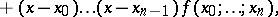where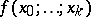are the divided differences of order; it was treated by I. Newton in 1687. Formula (1) is called Newton's interpolation formula for unequal differences. When theare equidistant, that is, if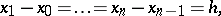then by introducing the notation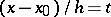and expressing the divided differencesin terms of the finite differences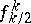according to the formula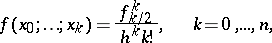one obtains a way of writing the polynomial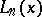in the form(2)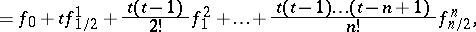which is called Newton's interpolation formula for forward interpolation. If the same change of variables is made in the interpolation polynomialwith nodes, where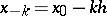,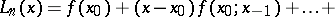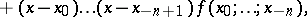then one obtains Newton's interpolation formula for backward interpolation: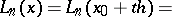(3)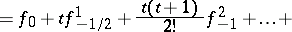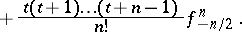Formulas (2) and (3) are convenient for computing tables of a given functionif the pointis at the beginning or the end of the table, since in this case the addition of one or several nodes caused by the wish to increase the accuracy of the approximation does not lead to a repetition of the whole work done as in computations with Lagrange's formula.

How to Cite This Entry:
Newton interpolation formula. Encyclopedia of Mathematics. URL: http://encyclopediaofmath.org/index.php?title=Newton_interpolation_formula&oldid=15016
This article was adapted from an original article by M.K. Samarin (originator), which appeared in Encyclopedia of Mathematics - ISBN 1402006098. See original article